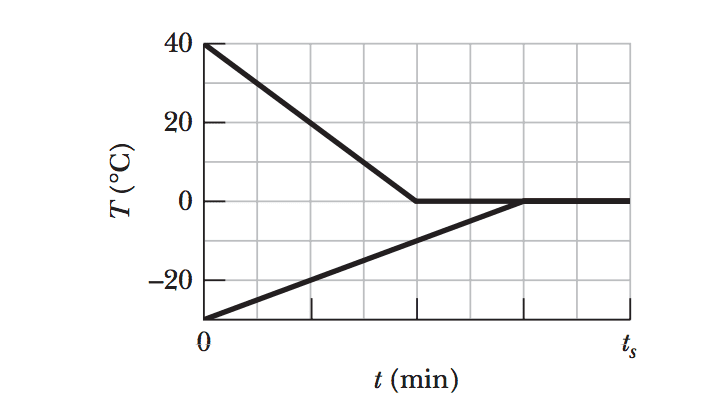# How much water is converted to ice?

## Homework Statement

A 0.530 kg sample of liquid water and a sample of ice are placed in a thermally insulated container. The container also contains a device that transfers energy as heat from the liquid water to the ice at a constant rate P, until thermal equilibrium is reached. The temperatures T of the liquid water and the ice are given in Fig. 18-35 as functions of time t; the horizontal scale is set by ts 80.0 min. (a) What is rate P? (b) What is the initial mass of the ice in the container? (c) When thermal equilibrium is reached, what is the mass of the ice produced in this process?

Q = mcΔT[/B]
P = Q/t

## The Attempt at a Solution

Hi, I already know the answer to all three parts, but my question is this: Why, for part (C), when finding the mass of water that turns into ice, do I set the total energy transfer (P*ts = 37W/s * 4800s = 177,600 J equal to the mass of water turned into ice (mw*LF)? Shouldn't some of this total energy go into lowering the temperature of the water first?